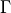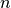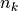# 11.2.1.3. astroML.density_estimation.knuth_bin_width¶

astroML.density_estimation.knuth_bin_width(data, return_bins=False, disp=True)[source]

Deprecated since version 0.4: The knuth_bin_width function is deprecated and may be removed in a future version. Use astropy.stats.knuth_bin_width instead.

Return the optimal histogram bin width using Knuth’s rule [Rc52d530680fe-1]

Parameters
dataarray-like, ndim=1

observed (one-dimensional) data

return_binsbool (optional)

if True, then return the bin edges

Returns
dxfloat

optimal bin width. Bins are measured starting at the first data point.

binsndarray

bin edges: returned if return_bins is True

Notes

The optimal number of bins is the value M which maximizes the functionwhereis the Gamma function,is the number of data points,is the number of measurements in bin.